# NEET Physics Kinetic Theory of Gases Questions Solved

An isolated system

(A) is a specified region where transfers of energy and mass takes place

(B) is a region of constant mass and only energy is allowed to close the boundaries

(C) is one in which mass within the system is not necessarily constant.

(D) cannot transfer either energy of mass to or from the surroundings.

Complete Question Bank + Test Series
Complete Question Bank

Difficulty Level: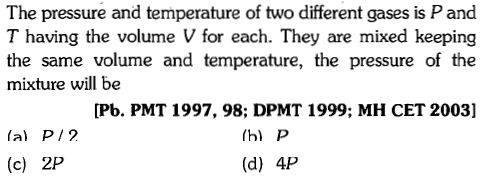Complete Question Bank + Test Series
Complete Question Bank

Difficulty Level: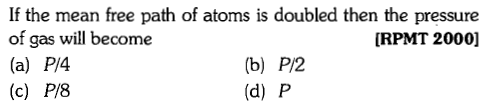Complete Question Bank + Test Series
Complete Question Bank

Difficulty Level: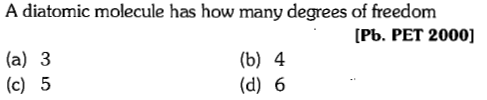Complete Question Bank + Test Series
Complete Question Bank

Difficulty Level: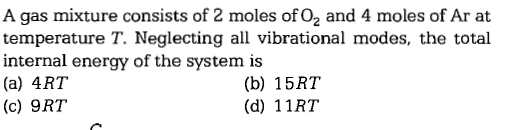Complete Question Bank + Test Series
Complete Question Bank

Difficulty Level:

Which of the following relations is correct for root mean square speed of a gas at temperature T?

(A)     (B)

(C)     (D)

Where k= Bolzmann constant,

m= mass of a molecule.

Complete Question Bank + Test Series
Complete Question Bank

Difficulty Level: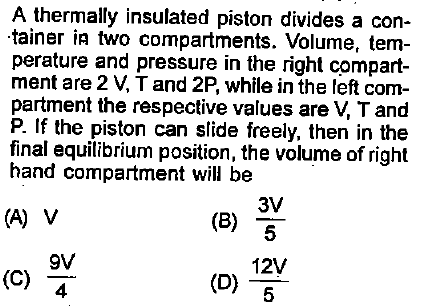Complete Question Bank + Test Series
Complete Question Bank

Difficulty Level: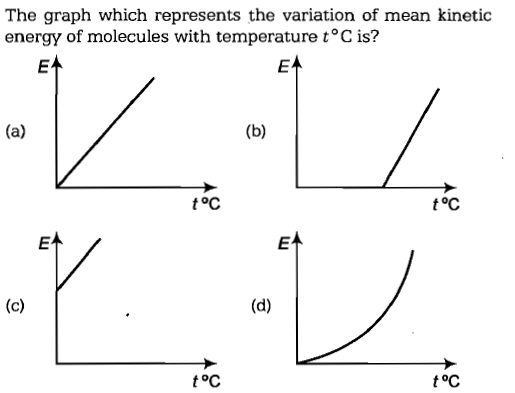Complete Question Bank + Test Series
Complete Question Bank

Difficulty Level: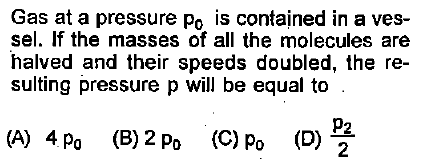Complete Question Bank + Test Series
Complete Question Bank

Difficulty Level:

${V}_{rms},{V}_{av},{V}_{mp}$ are rrot mean square, averageand most probable speeds of molecules of a gas obeying Maxwellian velocity distribution. Which of the following statements is correct:

1. ${V}_{rms}<{V}_{av}<{V}_{mp}$

2. ${V}_{rms}>{V}_{av}>{V}_{mp}$

3.

4. ${V}_{avg}>{V}_{rms}<{V}_{mp}$